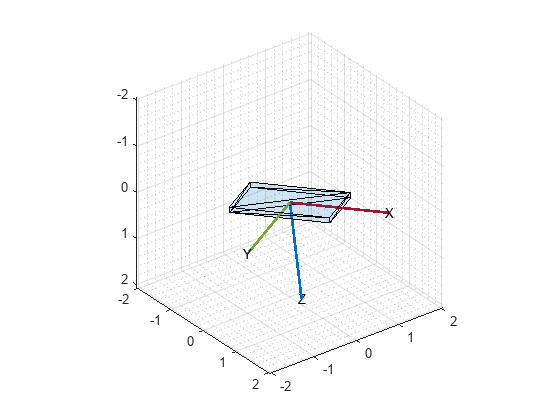# Estimate Phone Orientation Using Sensor Fusion

MATLAB Mobile™ reports sensor data from the accelerometer, gyroscope, and magnetometer on Apple or Android mobile devices. Raw data from each sensor or fused orientation data can be obtained. This examples shows how to compare the fused orientation data from the phone with the orientation estimate from the `ahrsfilter` (Navigation Toolbox) object.

### Read the Accelerometer, Gyroscope, Magnetometer, and Euler Angles

Read the logged phone sensor data. The MAT-file `samplePhoneData.mat` contains sensor data logged on an iPhone at a 100 Hz sampling rate. To run this example with your own phone data, refer to Sensor Data Collection with MATLAB Mobile or MATLAB Online.

```matfile = 'samplePhoneData.mat'; SampleRate = 100; % This must match the data rate of the phone. [Accelerometer, Gyroscope, Magnetometer, EulerAngles] ... = exampleHelperProcessPhoneData(matfile);```

### Convert to North-East-Down (NED) Coordinate Frame

MATLAB Mobile uses the convention shown in the following image. To process the sensor data with the `ahrsfilter` object, convert to NED, a right-handed coordinate system with clockwise motion around the axes corresponding to positive rotations. Swap the x- and y-axis and negate the z-axis for the various sensor data. Note that the accelerometer readings are negated since the readings have the opposite sign in the two conventions.```Accelerometer = -[Accelerometer(:,2), Accelerometer(:,1), -Accelerometer(:,3)]; Gyroscope = [Gyroscope(:,2), Gyroscope(:,1), -Gyroscope(:,3)]; Magnetometer = [Magnetometer(:,2), Magnetometer(:,1), -Magnetometer(:,3)]; qTrue = quaternion([EulerAngles(:,3), -EulerAngles(:,2), EulerAngles(:,1)], ... 'eulerd', 'ZYX', 'frame');```

### Correct Phone Initial Rotation

The phone may have a random rotational offset. Without knowing the offset, you cannot compare the `ahrsfilter` object and the phone results. Use the first four samples to determine the rotational offset, then rotate the phone data back to desirable values.

```% Get a starting guess at orientation using ecompass. No coefficients % required. Use the initial orientation estimates to figure out what the % phone's rotational offset is. q = ecompass(Accelerometer, Magnetometer); Navg = 4; qfix = meanrot(q(1:Navg))./meanrot(qTrue(1:Navg)); Orientation = qfix*qTrue; % Rotationally corrected phone data.```

### Tune the AHRS Filter

To optimize the noise parameters for the phone, tune the `ahrsfilter` object. The parameters on the filter need to be tuned for the specific IMU on the phone that logged the data in the MAT-file. Use the `tune` (Navigation Toolbox) function with the logged orientation data as ground truth.

```orientFilt = ahrsfilter('SampleRate', SampleRate); groundTruth = table(Orientation); sensorData = table(Accelerometer, Gyroscope, Magnetometer); tc = tunerconfig('ahrsfilter', "MaxIterations", 30, ... 'ObjectiveLimit', 0.001, 'Display', 'none'); tune(orientFilt, sensorData, groundTruth, tc);```

### Fuse Sensor Data With Filter

Estimate the device orientation using the tuned `ahrsfilter` object.

```reset(orientFilt); qEst = orientFilt(Accelerometer,Gyroscope,Magnetometer);```

### Plot Results

Plot the Euler angles for each orientation estimate and the quaternion distance between the two orientation estimates. Quaternion distance is measured as the angle between two quaternions. This distance can be used as an error metric for orientation estimation.

```numSamples = numel(Orientation); t = (0:numSamples-1).'/SampleRate; d = rad2deg(dist(qEst, Orientation)); figure plot(t, eulerd(qEst, 'ZYX', 'frame')) legend yaw pitch roll title('ahrsfilter Euler Angles') ylabel('Degrees') xlabel('Time (s)')``````figure plot(eulerd(Orientation, 'ZYX', 'frame')) legend yaw pitch roll title('Phone Euler Angles') ylabel('Degrees') xlabel('Time (s)')``````figure plot(t, d) title('Orientation Error') ylabel('Degrees') xlabel('Time (s)') % Add RMS error rmsval = sqrt(mean(d.^2)); line(t, repmat(rmsval,size(t)),'LineStyle','-.','Color','red'); text(t(1),rmsval + 0.7,"RMS Error = " + rmsval,'Color','red')```Use `poseplot` to view the orientation estimates of the phone as a 3-D rectangle.

```figure pp = poseplot("MeshFileName", "phoneMesh.stl"); for i = 1:numel(qEst) set(pp, "Orientation", qEst(i)); drawnow end```